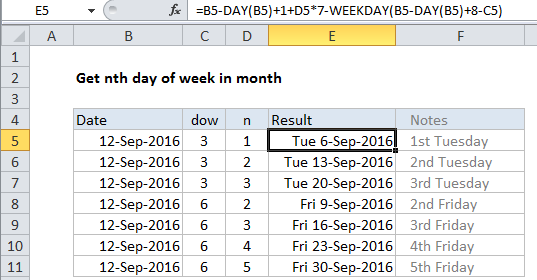## Excel Office

Excel How Tos, Tutorials, Tips & Tricks, Shortcuts

# How to calculate nth day of week in month in Excel

To get the nth day if week in a month (i.e. the first Tuesday, third Tuesday, forth Thursday, etc.) you can use a formula based on the DAY and WEEKDAY functions.

## Formula

`=date-DAY(date)+1+n*7-WEEKDAY(date-DAY(date)+8-dow)`Note:  Day of week is abbreviated “dow” in the generic form of the formula. This is a number between 1 (Sunday) and 7 (Saturday) which can be changed as needed.

Worked Example:   Generate series of dates by weekends in Excel

## Explanation

In the example shown, the formula in E5 is:

`=B5-DAY(B5)+1+D5*7-WEEKDAY(B5-DAY(B5)+8-C5)`

### How this formula works

First, this formula determines a “start date”. The “first of month” is calculated with the DAY function here:

`=B5-DAY(B5)+1`

Then  n * 7 days to the first of the month, to get a start date n weeks from the first of the month. In the generic form of the formula, n represents “nth”.

Worked Example:   Conditional formatting gantt chart weekends in Excel

The next step is to calculate the adjustment required to reach the final result. The adjustment is calculated using WEEKDAY and DAY:

`WEEKDAY(B5-DAY(B5)+8-C5)`

Inside WEEKDAY, the first of the month is again calculated, then 8 days are added and the value for dow (day of week) is subtracted.

Worked Example:   WEEKDAY function: Description, Usage, Syntax, Examples and Explanation

Finally the calculated adjustment is subtracted from original start date to get the final result.